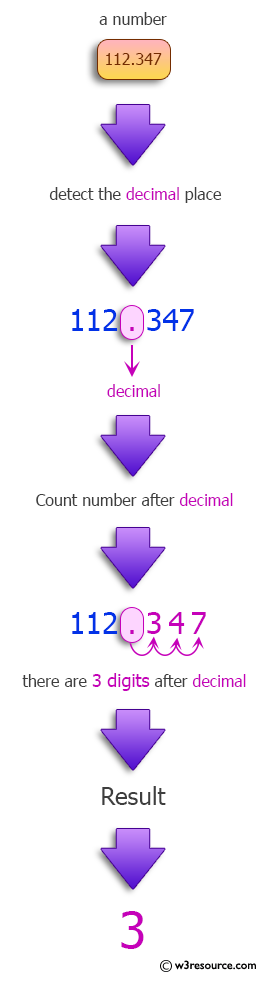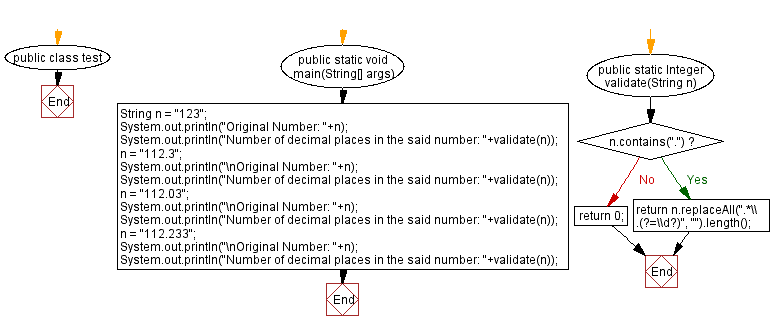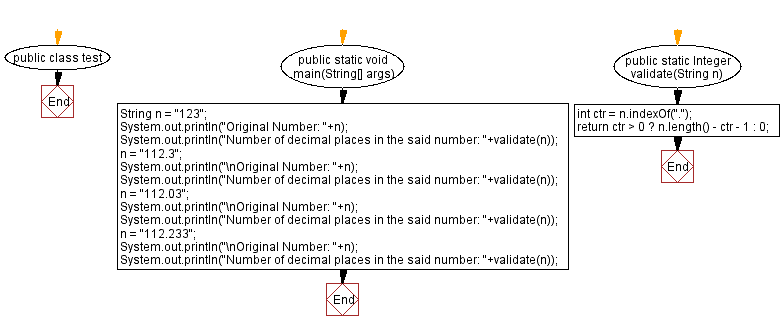﻿ Java: Count the number of decimal places in a given number# Java programming Exercises: Count the number of decimal places in a given number

## Java Regular Expression: Exercise-17 with Solution

Write a Java program to count the number of decimal places in a given number.

Sample Solution-1:

Java Code:

``````public class test {

public static void main(String[] args) {
String n = "123";
System.out.println("Original Number: "+n);
System.out.println("Number of decimal places in the said number: "+validate(n));
n = "112.3";
System.out.println("\nOriginal Number: "+n);
System.out.println("Number of decimal places in the said number: "+validate(n));
n = "112.03";
System.out.println("\nOriginal Number: "+n);
System.out.println("Number of decimal places in the said number: "+validate(n));
n = "112.233";
System.out.println("\nOriginal Number: "+n);
System.out.println("Number of decimal places in the said number: "+validate(n));
}

public static Integer validate(String n) {
if(n.contains("."))
return n.replaceAll(".*\\.(?=\\d?)", "").length();
return 0;
}
}
```
```

Sample Output:

```Original Number: 123
Number of decimal places in the said number: 0

Original Number: 112.3
Number of decimal places in the said number: 1

Original Number: 112.03
Number of decimal places in the said number: 2

Original Number: 112.233
Number of decimal places in the said number: 3
```

Pictorial Presentation:Flowchart :Sample Solution-2:

Java Code:

``````public class test {

public static void main(String[] args) {
String n = "123";
System.out.println("Original Number: "+n);
System.out.println("Number of decimal places in the said number: "+validate(n));
n = "112.3";
System.out.println("\nOriginal Number: "+n);
System.out.println("Number of decimal places in the said number: "+validate(n));
n = "112.03";
System.out.println("\nOriginal Number: "+n);
System.out.println("Number of decimal places in the said number: "+validate(n));
n = "112.233";
System.out.println("\nOriginal Number: "+n);
System.out.println("Number of decimal places in the said number: "+validate(n));
}

public static Integer validate(String n) {
int ctr = n.indexOf(".");
return ctr > 0 ? n.length() - ctr - 1 : 0;
}
}
```
```

Sample Output:

```Original Number: 123
Number of decimal places in the said number: 0

Original Number: 112.3
Number of decimal places in the said number: 1

Original Number: 112.03
Number of decimal places in the said number: 2

Original Number: 112.233
Number of decimal places in the said number: 3
```

Flowchart :Java Code Editor:

Next: Validate a personal identification number (PIN).

What is the difficulty level of this exercise?

Test your Programming skills with w3resource's quiz.

﻿

## Java: Tips of the Day

Split Java String by New Line

```String lines[] = string.split("\\r?\\n");
```

There's only really two newlines (UNIX and Windows) that you need to worry about.

Ref: https://bit.ly/3dHk7ip